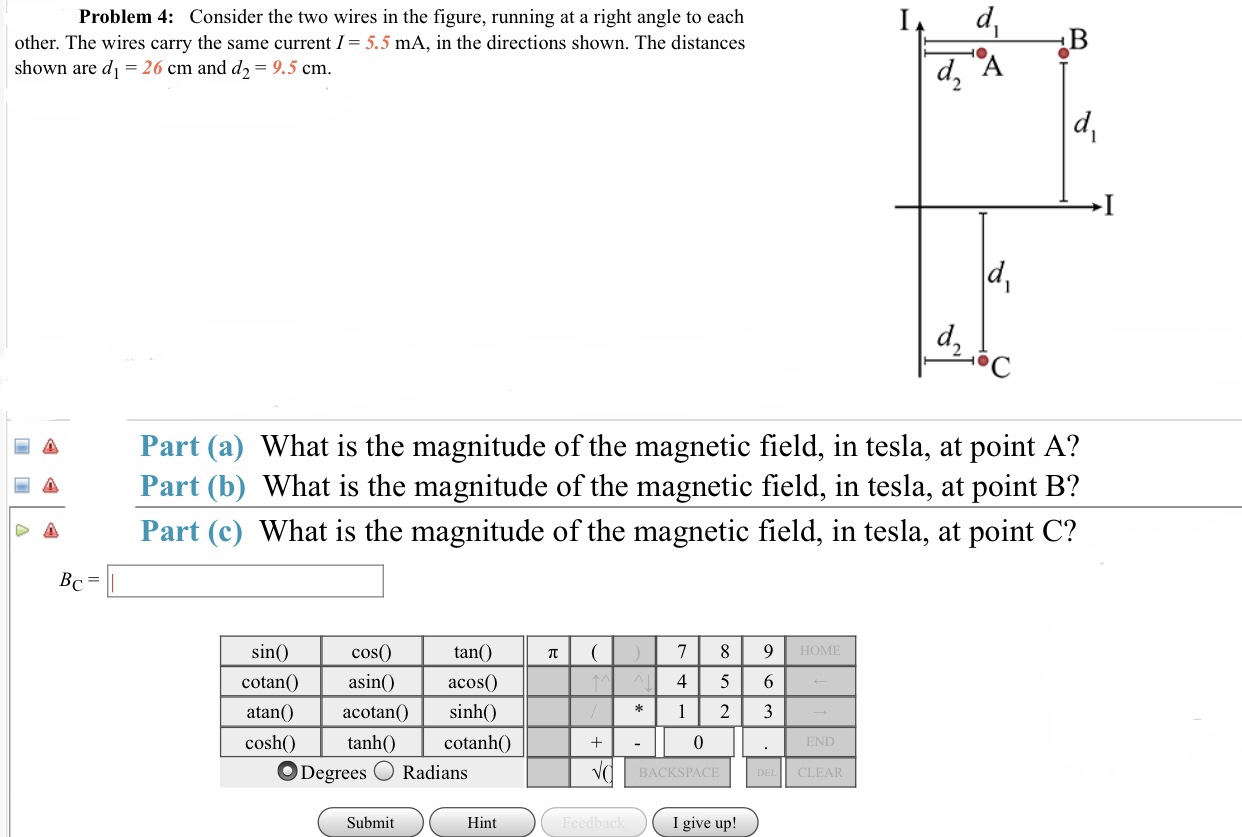# Problem 4: Consider the two wires in the figure, running at a right angle to eachother. The wires carry the same current I= 5.5 mA, in the directions shown. The distanceshown are d 26 cm and d2-9.5 cma Part (a) What is the magnitude of the magnetic field, in tesla, at point A?A Part (b) What is the magnitude of the magnetic field, in tesla, at point B?Δ Part (c) What is the magnitude of the magnetic field, in tesla, at point C?tan()cos(0asin()atan() acotan sinh()sinHOMEcotan asind acos4 5 6coshcotanhO0O Degrees O RadiansBACKSPACECLEARSubmitHintI give up!

Question
542 viewshelp_outlineImage TranscriptioncloseProblem 4: Consider the two wires in the figure, running at a right angle to each other. The wires carry the same current I= 5.5 mA, in the directions shown. The distances hown are d 26 cm and d2-9.5 cm a Part (a) What is the magnitude of the magnetic field, in tesla, at point A? A Part (b) What is the magnitude of the magnetic field, in tesla, at point B? Δ Part (c) What is the magnitude of the magnetic field, in tesla, at point C? tan() cos(0 asin() atan() acotan sinh() sin HOME cotan asind acos 4 5 6 cosh cotanhO 0 O Degrees O Radians BACKSPACE CLEAR Submit Hint I give up! fullscreen
check_circle

Step 1

(a)

The magnitude of the magnetic field at point A is,

Step 2

(b)

The magnitude of the magnetic field at point B is,

Step 3

(c)

The magnitude of the magnetic...

### Want to see the full answer?

See Solution

#### Want to see this answer and more?

Solutions are written by subject experts who are available 24/7. Questions are typically answered within 1 hour.*

See Solution
*Response times may vary by subject and question.
Tagged in

### Electromagnetic Induction